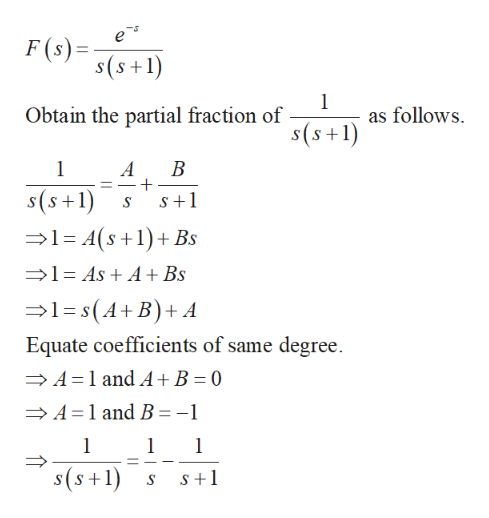# Find f(t).ℒ−1 {e-s/(s(s+1))}f(t)=____________________+(_____________________)U(t-________)

Question
4 views

Find f(t).

−1 {e-s/(s(s+1))}

f(t)=____________________+(_____________________)U(t-________)

check_circle

Step 1

Recall the following facts.

Step 2

Take the partial fracti...help_outlineImage Transcriptionclosee F (s)= s(s+1 Obtain the partial fraction of as follows s(s+1) A В s(s+1) S+1 1-A(s1 Bs 1-AsA + Bs 1-s(A+B) + A Equate coefficients of same degree A 1 and A+ B = 0 A 1 and B = -1 1 1 s(s+1) S+1 fullscreen

### Want to see the full answer?

See Solution

#### Want to see this answer and more?

Solutions are written by subject experts who are available 24/7. Questions are typically answered within 1 hour.*

See Solution
*Response times may vary by subject and question.
Tagged in